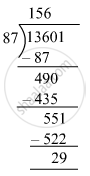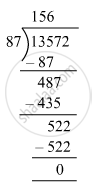# What least number must be subtracted from 13601 to get a number exactly divisible by 87? - Mathematics

Fill in the Blanks

What least number must be subtracted from 13601 to get a number exactly divisible by 87?

#### Solution

First, we will divide 13601 by 87.Remainder = 29
So, 29 must be subtracted from 13601 to get a number exactly divisible by 87.
i.e., 13601 − 29 = 13572
Now, we have:∴ 29 must be subtracted from 13601 to make it divisible by 87.

Is there an error in this question or solution?

#### APPEARS IN

RS Aggarwal Class 6 Mathematics
Chapter 3 Whole Numbers
Exercise 3E | Q 8 | Page 56